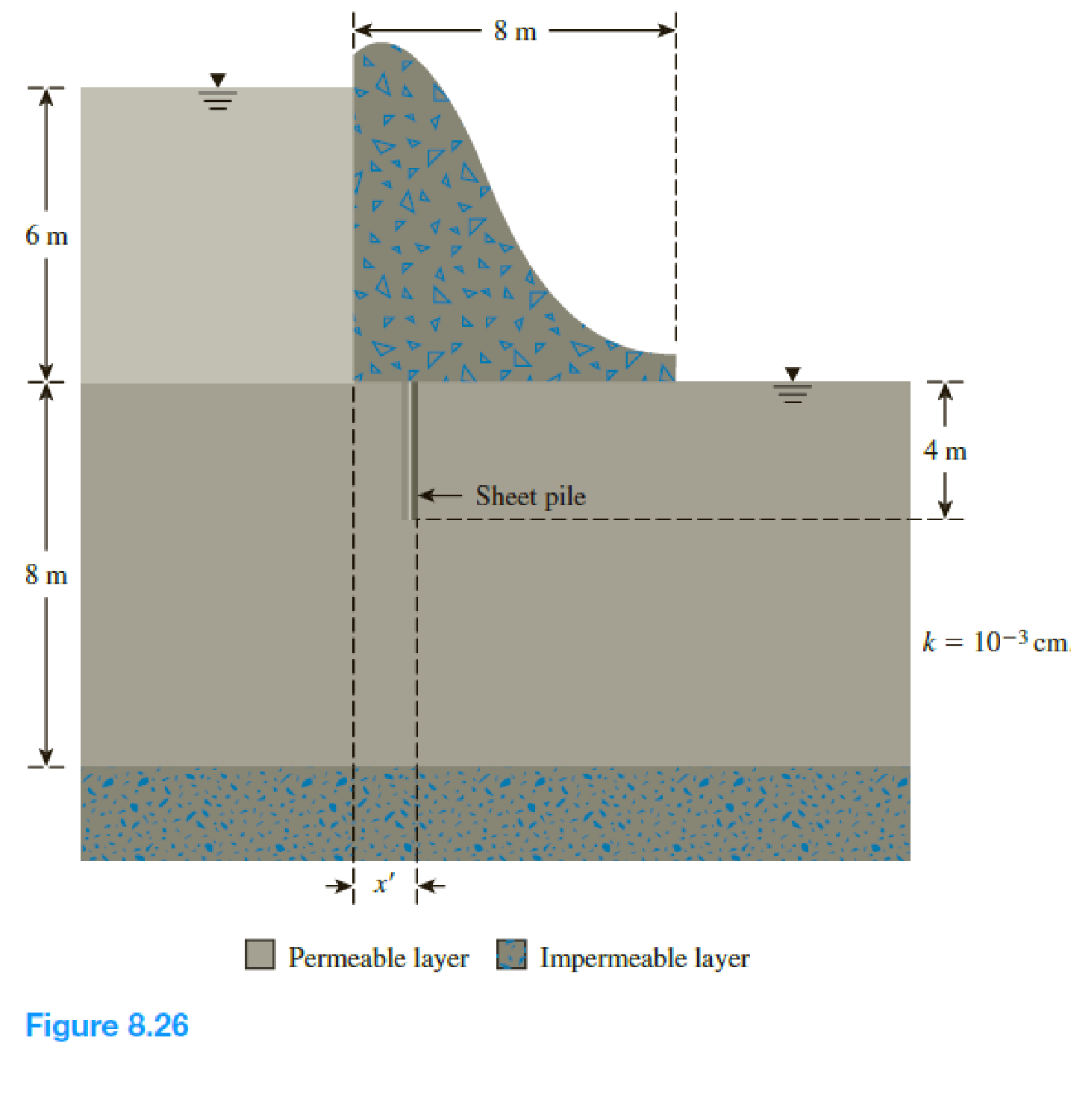Chapter 8, Problem 8.7PPrinciples of Geotechnical Enginee...

9th Edition
Braja M. Das + 1 other
ISBN: 9781305970939

Solutions

Chapter
SectionPrinciples of Geotechnical Enginee...

9th Edition
Braja M. Das + 1 other
ISBN: 9781305970939
Textbook Problem

For the weir shown in Figure 8.26, calculate the seepage in the permeable layer in m3/day/m for (a) x′ = 1 m and (b) x′ = 2 m. Use Figure 8.13.(a)

To determine

Find the seepage in the permeable layer in m3/day/m for x=1m.

Explanation

Given information:

The hydraulic conductivity of the permeable soil layer k is 103cm/sec.

The height of the water level H is 6 m.

The depth of penetration of the sheet pile S is 4 m.

The thickness of the permeable soil layer T is 8 m.

The value of width B is 8 m.

Calculation:

Determine the ratio of S/T.

Substitute 4 m for S and 8 m for T.

S/T=48=0.5

Determine the value of x using the relation

x=bx=B2x

Substitute 8 m for B and 1 m for x.

x=821=3m

Determine the ratio of xb.

Substitute 3 m for x and (82)m for b.

xb=38/2=0.75

Determine the ratio of bT.

Substitute (82)m for b and 8 m for T

(b)

To determine

Find the seepage in the permeable layer in m3/day/m for x=2m.

Still sussing out bartleby?

Check out a sample textbook solution.

See a sample solution

The Solution to Your Study Problems

Bartleby provides explanations to thousands of textbook problems written by our experts, many with advanced degrees!

Get Started

How can you protect against shoulder surfing?

Principles of Information Security (MindTap Course List)

What is a journeyperson?

Precision Machining Technology (MindTap Course List)

Explain how to use a micrometer to measure cam lobe wear.

Automotive Technology: A Systems Approach (MindTap Course List)

What is a cost-benefit analysis?

Management Of Information Security

What characteristics of the weld bead do the weaving of the electrode cause?

Welding: Principles and Applications (MindTap Course List)

The _____ web design approach adapts the layout of the website to fit the screen on which it is displayed.

Enhanced Discovering Computers 2017 (Shelly Cashman Series) (MindTap Course List)